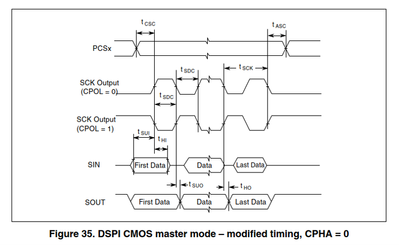# MPC5777C SPI modified timing

cancel
Showing results for
Show  only  | Search instead for
Did you mean:

## MPC5777C SPI modified timing

323 ViewsContributor I

Hello,

We have a question regarding the SPI SOUT timing. The behaviour of SOUT doesn't match with the values inside of the MPC5777C datasheet.

We use the SPI with modified timing at 30 MHz with the following settings:

CPOL = 0, CPHA = 0, PCR[SCR] = 11b, t_sys = 16,67, f_sys = 60 MHz

Therefore according to p. 68 of the Datasheet t_SUO = max(23,67 ns) and t_HO = min(7,67 ns).Our first problem is, that we don't see a real difference between t_SUO and t_HO in Figure 35 (p. 69 MPC5777C Datasheet). As we understand it, the values t_HO and t_SUO are both referenced to the falling edge of the clock. To the rigth of the reference the value is positive and to the left the value is negative.

Our second problem is, that we measured t_HO = 1,8 ns (see DSPI_t_HO.jpg in the attachment) which is more than 5 ns below the minimum value. There is no difference between the different DSPI modules. All Modules have the same behaviour with t_HO between 0-2ns. If we consider t_HO and t_SUO the same t_SUO = 1-2 ns aswell. With the assumption t_SUO != t_HO the next possible value for t_SUO would be 34 ns, which is higher than the maximum value.

Edit:

What is the difference between t_SUO and t_HO?

Are we correct with our assumptions for our first problem?

Can you confirm the values we measured?

Did we measure the timings wrong?